## book reviewsFOUNDATIONSADVANCES
ISSN: 2053-2733
Volume 76| Part 2| March 2020| Pages 269-272

An Introduction to Clifford Algebras and Spinors. By Jayme Vaz Jr and Roldão da Rocha Jr. Oxford University Press, 2019. Paperback, pp. 256. Price GBP 34.99. ISBN 9780198836285.

aInternational Christian University, Osawa 3-10-2, Mitaka-shi, Tokyo, 181-8585, Japan
*Correspondence e-mail: hitzer@icu.ac.jp

An Introduction to Clifford Algebras and Spinors by two Brazilian physicists, Jayme Vaz Jr and Roldão da Rocha Jr of IMECC, Universidade Estudual de Campinas and CMCC – Universidade Federal do ABC, was first published by Oxford University Press in hardcover in 2016 and republished in 2019 as a paperback. The 256-page book begins with a sweeping preface that relates the historic works on unifying geometric and algebraic operations by celebrities like G. Leibnitz, J.-R. Argand, C. F. Gauss, R. W. Hamilton, H. G. Grassmann, W. K. Clifford, E. Cartan, W. Pauli, P. Dirac etc. For crystallographers it may be of interest to know that J. G. Grassmann (Justus G. was the father of Hermann G. Grassmann) originally introduced the characterization of crystal planes by orthogonal vectors, now commonly denoted with Miller indices [see Erhard Scholz in Schubring (1996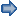), pp. 37–46]. J. G. Grassmann's work, including his mathematical school textbooks, provided H. G. Grassmann with fertile ideas for his new concepts of algebra, solely defined by the relations of its elements, from which G. Peano distilled the modern concept of vectors. Grassmann's pioneering approach was so far ahead of its time that only a few bright minds (like R. W. Hamilton, F. Klein and S. Lie) recognized its genius during his lifetime, late in Grassmann's life. But the young Cambridge-educated genius W. K. Clifford was truly exceptional, and published in 1878 (one year after Grassmann's death) his seminal paper Applications of Grassmann's Extensive Algebra' in Am. J. Math. It elegantly unified the earlier works of Hamilton on quaternions and Grassmann's metric-free algebra of extension to geometric algebras (now known as Clifford algebras), by simply adding in the Clifford (or geometric) product the inner product of vectors (necessary for measurements) and the outer product of Grassmann.

The authors began to develop their subject 20 years ago in the form of lecture notes in several university courses and have plenty of their own publications on the subject.

The text is divided into seven chapters, each completed with a final subsection full of exercises and recommendations for further reading. Readers will have to work their way through the subject-specific notation, for which the well-ordered seven-page Appendix B List of Symbols' provides essential support. References (7 pp.) and an index (4 pp.) complete the backmatter. The book may therefore in parts be used for lecture courses to graduate students, or alternatively for self-study and as a launch pad for further research.

It is of interest to anybody who wants to understand a modern description of spinors in Clifford algebra language. The level is clearly graduate student or above. It will be easiest to read for physicists and mathematicians, but crystallographers with sufficient mathematical background in linear algebra, tensor algebra and group theory should find it accessible. For readers who want an introduction to Clifford algebras, which will make reading this introduction to spinors easier, I would recommend the two college-level textbooks by MacDonald (2011, 2012) and the recent Springer Brief by Xambó-Descamps (2018).

In Preliminaries' (Chapter 1) vectors are introduced with eight axioms for addition and scalar multiplication (scalars are here taken as elements of a field, e.g. real and complex numbers and quaternions). For quaternions left and right multiplication must be distinguished. Then the dual vector space of linear functionals mapping vectors into scalars equipped with a covector basis is defined. Covariant and contravariant transformations are distinguished and their practical use is demonstrated with two pages of examples in three-dimensional Euclidean space and for polynomials of degree less than or equal to 2. Consideration is given to the bidual, kernel and image of linear transformations, correlations (maps from vector to dual vector space) and bilinear functionals, and the distinction between quadratic and symplectic spaces. Special compact musical' notation is introduced for symmetric correlations (i.e. correlations and their inverse) and illustrated with an example in three-dimensional Euclidean space. Since this notation is quite particular, readers should take care not to skip over these preliminaries. Next, the tensor product of arbitrary numbers of vectors and covectors, tensor bases, symmetry, mixed bilinear functionals and transformations of tensors are reviewed and illustrated with examples from two and three dimensions, followed by the definition of direct sums of vector spaces. The preliminaries conclude by introducing tensor algebra (sums and products of tensors), grading of tensors, grade involution, and even and odd grade projection. Another involution is reversion, conjugation being the combination of it with grade involution. A set of eight exercises reinforces and extends the content covered in the preliminaries.

Chapter 2 on Exterior Algebra and Grassmann Algebra' reviews these two algebras and their relation to tensor algebra, ending with the Hodge isomorphism. After commenting on Grassmann's original work (1862), it defines permutations and the alternator operator (with the example of symmetric group S3), showing its application to covariant and contravariant tensors and for the definition of a matrix determinant. p-Vectors are defined as alternating covariant tensors of order p, similarly p-covectors, their spaces being denoted with the famous capital Greek lambda symbol, and their dimensions being considered. The alternator is applied to define (Definition 2.1) and study the exterior product of p-vectors. A variety of definitions and more advanced literature on the subject are given as Observation' (p. 27). Then notions of the basis for p-vector spaces, the notational sum convention and maximum dimension n-vectors (pseudoscalars) are introduced. The combination of the direct sum of all p-vector spaces with the exterior product leads to exterior algebras (Definition 2.2) for both vectors and covectors. Examples in three and four dimensions and as an application to solving a linear system of equations are given. It is observed that the exterior algebra can be obtained as a quotient in tensor algebra (a consequence of the theorem on p. 35), starting with elementary notions of equivalence relations and ideals. The ideal used is the kernel of the alternator. Next the notions of left and right contractions (inner product) by covectors are defined, studied and generalized to p-covectors together with an example in four dimensions. Vector space orientation is defined by the choice of a pseudoscalar, which in turn serves to define quasi-Hodge isomorphisms (two examples show its application). Slightly deviating from Grassmann's original (he used the Hodge star operator called in German Ergänzung), the authors define the regressive product based on the quasi-Hodge isomorphism. By endowing exterior algebra with an extension of a metric bilinear functional (also called metric) to all of the exterior algebra, the authors define Grassmann algebra (Definition 2.3), and an example is given for three dimensions. The Hodge isomorphism is defined between p-vector spaces of dimensions p and np, and its relation with the quasi-Hodge isomorphism is made explicit. Chapter 2 is rounded off by advising additional reading and providing ten exercises including Plücker coordinates and the cross product of Gibbs–Heaviside vector algebra.

The following three chapters are all on (real and complex) Clifford or geometric algebra(s).

Chapter 3 provides their definition, the relation to tensor algebra and Grassmann algebra. The definition (Definition 3.1) is that of generation by elements of a quadratic vector space (with non-degenerate metric), with unity, and the anti-commutator resulting in the metric. Next, isomorphism-based universality of a Clifford algebra is defined. This is the case for dimension 2n (Theorem 3.1). The existence is shown (Theorem 3.2) and examples for n = 1 and 3 dimensions follow. Like exterior algebra, Clifford algebra can also be obtained as a quotient of tensor algebra, the relevant ideal being the tensor product of a vector minus its metric times unity, as proposed in 1954 by Chevalley. Observations on notation (p. 64) are given (important for readers beyond this point) and the universality of the tensor quotient definition is shown (Theorem 3.3). Dimension and vector space(!) isomorphisms with exterior and Grassmann algebra are given, and the computational preference for orthogonal bases is explained. The center of a Clifford algebra and isomorphisms of grade involution, reversion and (Clifford) conjugation are introduced, with obvious relations to corresponding notions in tensor algebra. An example computes the reversion of a product of two (simple) mono-grade Clifford algebra elements (p-vectors). The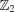grading is clarified, the general grade structure of the product of two simple elements of grades p, q is shown, and the extension of the metric to p-vectors and norm definitions are added. Examples illustrate Clifford algebras Cl1,2, Cl3,0 and Cl3,1. Two theorems on properties of non-universal Clifford algebras follow. Then, in the context of Grassmann algebra creation and annihilation operators (note the relation to quantum field theory) based on exterior product and contraction are defined, studied and combined to two interrelated Clifford mappings. Next follows a subsection on the mutual relation between Clifford and Grassmann algebras with observations on the exterior product, and contractions in Clifford algebra. To assist readers with recommended additional readings a subsection on notation is added. Finally, seven instructive exercises round off the chapter.

Chapter 4 extensively treats the Classification and Representation of the Clifford Algebras' with many explicit examples of representations, isomorphisms, relations to quaternions and real and complex matrix algebras. It begins with an introduction to the alternating tensor product, a set of theorems on the structure of Clifford algebras for combining two such algebras, complexification of a Clifford algebra, and elementary isomorphisms. Second, the task of classification is addressed starting with low-dimensional cases and for the general case isomorphisms to square matrix algebras over real and complex numbers and quaternions are employed. Up to dimension n = 7, exhaustive isomorphism listings are provided. The result is that Clifford algebras are determined by the signature parameters p, q of Clp,q, i.e. by the difference r = p - q mod 8, where it matters if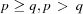or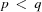. The results are summarized in table form (pp. 101–102) providing helpful reference information. Next, the notions of regular representation, idempotents, simple algebras and their relations are discussed and applied to Clifford algebra representations with examples in Cl2,0 and Cl3,0. Theorem 4.6 specifies the general product structure of primitive idempotents in Clifford algebras and the resulting group thus generated. Again, extensive examples for Cl0,7, Cl1,3 and Cl4,1 follow. Hermitian conjugation and transposition in the Clifford algebra context are discussed and expressed in a case-dependent way by products and involutions with an illustrative example in Cl4,1. Additional reading is recommended and three exercises with quaternionic matrix representation for Cl1,3, Dirac gamma matrices, and Weyl or chiral representation for complexified Cl1,3 and Majorana representation for Cl3,1 are given.

This is followed by Chapter 5 on Clifford Algebras and Associated Groups' beginning with orthogonal transformations, components of orthogonal groups and special orthogonal groups (det T = 1, T a transformation in the group), an example of O(1, 1), orthogonal symmetries and reflections. Then the Cartan–Dieudonné theorem that orthogonal transformations can be represented in Clifford algebra by reflections with respect to hyperplanes is proved in its weak version; the strong version shows that n reflections suffice (n = dim V). For a modern proof of the strong version the reader needs to look elsewhere [see e.g. Xambó-Descamps (2018)]. Next, Clifford–Lipschitz groups are discussed as subgroups of the invertible Clifford element group. Important tools are the adjoint representation and its twisted version. The latter in particular leads to elegant representations of the twisted Clifford–Lipschitz group [defined in equation (5.47)], the orthogonal and special orthogonal groups. As illustration the example Cl2,0 is studied. Further notable subgroups of Clifford–Lipschitz groups are pin groups (with group element norm = ±1) and spin groups (even grade group elements with norm = ±1). If the norms = +1, the groups are termed reduced with symbol index +. The twofold covering of Pin+ for O+ and Spin+ for SO+ are explained, and a theorem shows that Spin+(p, q) =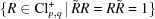, where Cl+ is the even subalgebra and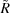the reverse of R. Examples are given for Spin+(1, 1) and Spin(3). Furthermore, the Lie algebras of the thus-introduced groups are discussed in Clifford algebra terms. For example, the commutator algebra of bivectors is found to be the Lie algebra of Spin+(p, q), and the example of Spin(3) is given. Then follows a study of conformal transformations in the context of Clifford algebras, beginning with plane Möbius transformations, conformal compactification resulting in O(p + 1, q + 1) as a twofold covering of the conformal group Conf(p, q). Attention is given to Möbius transformations in Minkowski spacetime (p = 1, q = 3), conformal transformations (with details listed in Table 5.1), and to the Lie algebra of the conformal group, generated by the bivectors in Cl2,4. The chapter closes with additional reading recommendations and five instructive exercises. We note that the two ingredients of the Cartan–Dieudonné theorem and conformal transformations in Clifford algebras famously led Hestenes & Holt (2007) to their comprehensive study of space groups in terms of three specific vector generators providing the necessary intelligence for the complete three-dimensional interactive Space Group Visualizer (https://spacegroup.info/, Hitzer & Perwass, 2010), that can be linked live to the online edition of Volume A of the International Tables for Crystallography.

The heart of the book really is the overlong Chapter 6 (64 pp.) on Spinors', which might have been better split into three chapters. At its beginning the authors clarify three common notions of spinors: algebraic (spin group in Clifford algebra), classical (with irreducible spin group representation) and spinor operators (using the even subalgebra of a Clifford algebra as representation space). One subsection is devoted to each of the three common notions of spinors. In the subsection on algebraic spinors, the classification depends on the value of pq mod 8, the results being summarized in Table 6.1 (real case) and Table 6.2 (complex case). The subsection on classical spinors relies on the classification of real even subalgebras (Table 6.3). Here as well the classification depends on pq mod 8, and the results are listed in Table 6.4 (real case) and Table 6.5 (complex case). A more extensive subsection is devoted to spinor operators as elements of the graded irreducible representation space of Clp,q. Six examples help to make this notion more concrete. A generalization of spinor algebra published by Mosna et al. (2003) is reviewed in some detail, with key roles for the pseudoscalars of Clp,q, Clp0,q0 and Clp-p0, q-q0. Examples for Cl1,1, Cl3,0 and Cl4,1 are given. The next subsection provides a detailed comparison of the three different spinor definitions, where up to dimension n = 5 all spinors and spinor operators are listed in Table 6.6. It is found that the case restricted to complex Clifford algebras is much simpler and then all three spinor types can easily be summarized in two lines as in Table 6.7. Detailed examples for Cl3,0 and Cl1,3 close this subsection on comparisons. Next, the inner product in the space of algebraic spinors is discussed with a spinor structure map and two types of inner products in spinor space, depending on the use of reversion or Clifford conjugation. An example illustrates this for Cl1,3, and then the important physics case of charge conjugation is algebraically described in complexified Clifford algebras. A further subsection is devoted to the triality principle in Clifford algebras, basically drawing on material from Benn & Tucker (1987). For the eight-dimensional Euclidean space V, the space E is defined as the direct sum of V with its 16-dimensional spinor space S. E is endowed with a commutative, non-associative Chevalley product and an operator EE is constructed and shown to be an order-3 automorphism (Theorem 6.1). This can in particular be realized in octonion algebra (see Table 6.8) that can in turn be defined as a special product on the direct sum of real numbers and the seven-dimensional anti-Euclidean space. A further subsection concentrates on the treatment of pure spinors as defined by Cartan (1938). The Cartan equation vu = 0, where v is a 2r-dimensional complex vector, the spinor u a vector in the 2r-dimensional representation space of the spin group, means for u not equal to zero that v is isotropic. Example 6.4 thus employs the Witt decomposition for the real space with signature (p, q) to construct the maximal totally isotropic subspaces. Definition 6.4 then defines the general concept of a pure spinor in Cl(V, g). An alternative pure spinor characterization is specified in Theorem 6.2. A spinor space basis can always be composed of pure spinors. Then considerations follow of dual rotations (using the volume element of the maximal totally isotropic subspace of the complexified base space), Penrose flagpoles and a generalization of these flagpoles (via dual rotations). Considered next are Weyl spinors in Cl3,0, with definitions of dotted and undotted, covariant and contravariant spinors, respectively, based on idempotents involving the vector e3. A similar algebraic description is given for Weyl spinors in Cl0,3 isomorphic to a direct sum of quaternions. General elements R in Cl3,0 with R conjugate(R) = 1, hence elements of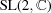, allow one to derive the transformation rules for Weyl spinors. The concept of paravectors (combinations of scalars and vectors) in Cl3,0 allows one to represent spacetime vectors, that can be generated by Weyl spinors, including the spacetime metric. Furthermore, paravectors of Cl4,1 can be generated from Cl3,0 as well, involving the Klein absolute of the real space with signature (2, 4). Next, twistors are discussed in two ways [following Keller (1997) on the one hand, and a generic alternative approach based on Cl4,1 paravectors on the other hand] as geometric multivectors (elements of the Dirac algebra Cl4,1). Finally, a quantum-physics-motivated spinor classification by bilinear covariants is thoroughly developed exclusively in the language of Clifford algebra. The chapter ends with additional reading recommendations and a set of five instructive exercises.

For comparison Appendix A gives the Standard Two-Component Spinor Formalism' of Weyl spinors, the four types of contravariant and covariant, dotted and undotted spinors, null flags, flagpoles and one page on supersymmetry algebra in conventional terms. This may greatly help readers familiar with theoretical physics and spinor theory to understand the correspondence between standard textbook formalism and the new comprehensive Clifford-algebra-based approach to spinors presented in great detail in this book.

The authors have taken on a gargantuan task of comprehensively casting spinor theory in the language of Clifford algebras, and based on their own research show how this opens up new insights and avenues of advancing this field. They make great efforts to introduce the historic literature and recommend further reading. Nevertheless, in one instance, I thought due credit should have been given for what the authors call `an amazing interpretation for a spinor in three-dimensional Euclidean space' (p. 163) that carries over to four-dimensional spacetime (p. 165). Basically, the spinor appears as a geometric rotation and dilation operator applied to a unit vector (that had been used to construct a primitive idempotent) resulting in the current density of quantum mechanics. This result can already be found in Hestenes' classic Spacetime Algebra [Hestenes (2015), as listed in the references, p. 235].

In conclusion, the book is written very thoroughly, enhances previous textbooks on the subject (e.g. Lounesto, 2001), comprehensively demonstrates that Clifford algebras are the ideal mathematical language for spinors, and comes with all the peripherals needed for teaching, self-study or for use as a reference text; it certainly is worth the money.

### ReferencesFOUNDATIONSADVANCES